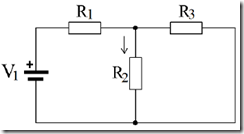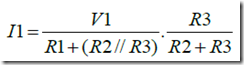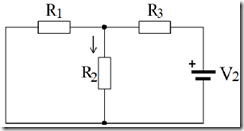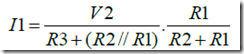" /> Theory Super Position | TN Elektro
Home > English > Theory Super Position

# Theory Super Position

The theory of superposition is used to analyze the circuit consisting of multiple voltage sources and prisoners. The voltage source can be either a voltage or current source itself. The theory of superposition facilitate determining the current on a branch with regard sources working one by one. The total current in the branches of an algebraic sum of the currents of each source with attention to the direction of flow.

When working on one source, then short circuited another source (for source voltage) and open linked to a current source. For more details see the circuit in figure 4.7 below.Figure 4.7 series resistor with a second voltage source

To calculate the current in R2 can be done by calculating the current caused by V1 and V2 are alternately later and the scores are added.
Step – step calculate the current in R2 is as follows:
1) Calculated currents by the voltage source V1 is I1, the equivalent circuit like Figure 4.8.Figure 4.8 equivalent circuit, when V2 short circuited

From the picture above the current equation:2) Calculated currents by the voltage source V2 is I2, the equivalent circuit as shown 4.9Figure 4.9 equivalent circuit, short circuited when V1

From the picture above the current equation:3) Calculate the current flowing in R2 is I, which is the sum of I1 and I2 (because the same direction), then I = I1 + I2